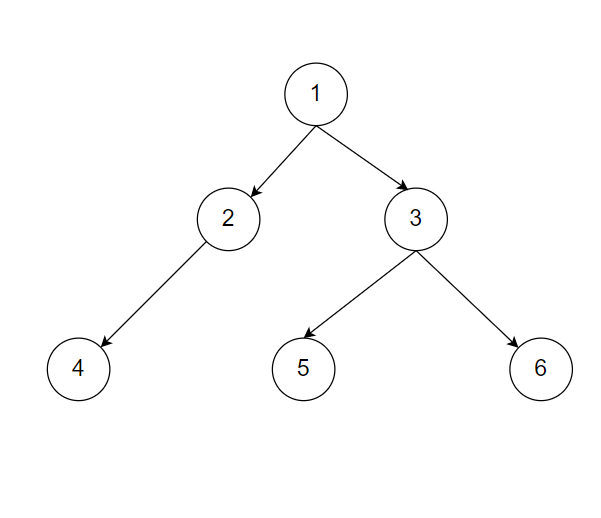# Find all Special Nodes.

Posted: 29 Mar, 2021
Difficulty: Moderate

## PROBLEM STATEMENT

#### In a binary tree, a special node is a node that is the only child of its parent. The root of the tree is not special because it does not have a parent node. Node ‘1’ is always the root of the binary tree. Return an array/list containing the values of all special nodes in the tree.

##### Example:
``````Let’s say you have a binary tree as follows:-
````````````Node 4 is a special node as it is the only child of node 2. Node 1 cannot be a special child as it does not have a parent. All the other nodes have a sibling i.e there parent has more than one child. Therefore return  as the answer.
``````
##### Note:
``````Return the array/list containing special nodes in any order.
``````
##### Input Format:
``````The first line of input contains an integer ‘T’ denoting the number of test cases.
The next ‘T’ lines represent the ‘T’ test cases.

The first line of input contains the elements of the tree in the level order form separated by a single space.
If any node does not have a left or right child, take -1 in its place. Refer to the example below.

Example:

Elements are in the level order form. The input consists of values of nodes separated by a single space in a single line. In case a node is null, we take -1 in its place.

For example, the input for the tree depicted in the below image would be :
```````````` 1
2 3
4 -1 5 6
-1 7 -1 -1 -1 -1
-1 -1
``````
##### Explanation :
``````Level 1 :
The root node of the tree is 1

Level 2 :
Left child of 1 = 2
Right child of 1 = 3

Level 3 :
Left child of 2 = 4
Right child of 2 = null (-1)
Left child of 3 = 5
Right child of 3 = 6

Level 4 :
Left child of 4 = null (-1)
Right child of 4 = 7
Left child of 5 = null (-1)
Right child of 5 = null (-1)
Left child of 6 = null (-1)
Right child of 6 = null (-1)

Level 5 :
Left child of 7 = null (-1)
Right child of 7 = null (-1)

The first not-null node (of the previous level) is treated as the parent of the first two nodes of the current level. The second not-null node (of the previous level) is treated as the parent node for the next two nodes of the current level and so on.

The input ends when all nodes at the last level are null (-1).
``````
##### Note :
``````The above format was just to provide clarity on how the input is formed for a given tree.

The sequence will be put together in a single line separated by a single space. Hence, for the above-depicted tree, the input will be given as:

1 2 3 4 -1 5 6 -1 7 -1 -1 -1 -1 -1 -1
``````
##### Output Format:
``````For each test case return the array/list containing all special nodes.
``````
##### Note
``````You do not need to print anything; it has already been taken care of. Just implement the function.
``````
##### Constraints:
``````1 <= T <= 10
1 <= N <= 10^3

Where ‘T’ is the number of test cases and ‘N’ is the number of nodes in the binary tree.

Time Limit: 1sec
``````Approach 1

We will start from the root and check if the node has one child, if yes then we will insert the child in the array/list ‘ans’. We will push all the children of the current node in the queue and iteratively visit all the children.

We will apply the algorithm as follows:-

1. Declare a queue ‘q’ which stores nodes whose children are not yet visited.
2. Declare ‘ans’ to store all the special nodes.
3. Insert node ‘1’ i.e root in the queue ‘q’.
4. While queue is not empty:-
• Store front of the queue in ‘u’
• Pop the front of the queue ‘q’.
• If ‘u’ has only a left or right child, insert that child in ‘ans’ as it is a special node.
• Insert all the children of ‘u’ into the queue ‘q’.
5. If ‘ans’ is empty, insert ‘-1’ in it.
6. Return ‘ans’.